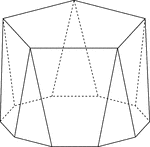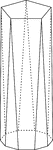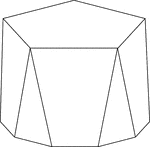Polyhedron With Pentagon Bases

Illustration of a pentagonal polyhedron that is formed by having two parallel congruent pentagonal bases…Polyhedron With Pentagon Bases

Illustration of a pentagonal polyhedron that is formed by having two parallel congruent pentagonal bases…Polyhedron With Pentagon Bases

Illustration of a pentagonal polyhedron that is formed by having two parallel congruent pentagonal bases…